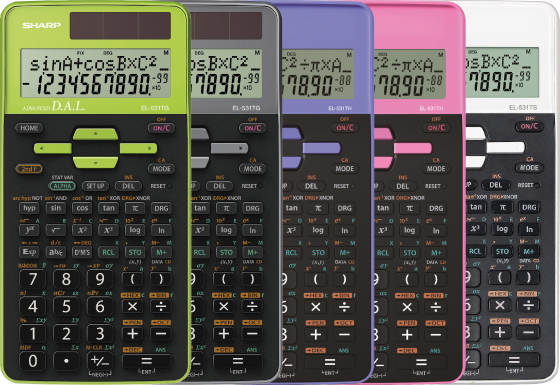## EL-531TH/TG

The EL-531TH and EL-531TG calculators perform 273 statistical, trigonometric and mathematical functions, including the possibility to browse and edit previous calculations. The large 2-line display has a 10+2 digits capacity. Both calculators are equipped with a hard cover, where you can choose battery only or dual power version and also up to five colors – green, pink, purple, white and gray.## Specification EL-531TH

• Built-In Functions: 273
• Entry System: Algebraic (D.A.L.)
• Power Source: Battery AAA × 1
• Dimensions and Weight: 161 × 80 × 15 mm, 115 g

Mathematical Functions

• Fractions

Memory

• Multi-Line Playback
• Memory Variables: 9

Notation and Decimal Places

• FSE Display (SCI, FIX, ENG)
• 3-Digit Punctuation

Statistics

• 1-Variable Statistics
• 2-Variable Statistics
• Regression Calculations: 6
• Mean, Standard Deviation
• nCr, nPr, n!
• Random Numbers

Trigonometric Functions

• Sin, Cos, Tan and Inverses
• Hyperbolic Functions
• Sexagesimal and Decimal Conversions
• Rectangular and Polar Coordinates Conversions

Number Systems

• Number Systems: DEC, BIN, OCT, HEX, PEN
• Logical Operation (AND, OR, NOT, NEG, XOR)

## Specification EL-531TG

• Built-In Functions: 273
• Entry System: Algebraic (D.A.L.)
• Power Source: Solar & Battery
• Dimensions and Weight: 161 × 80 × 15 mm, 110 g

Mathematical Functions

• Fractions

Memory

• Multi-Line Playback
• Memory Variables: 9

Notace a počet desetinných míst

• FSE Display (SCI, FIX, ENG)
• 3-Digit Punctuation

Statistics

• 1-Variable Statistics
• 2-Variable Statistics
• Regression Calculations: 6
• Mean, Standard Deviation
• nCr, nPr, n!
• Random Numbers

Trigonometric Functions